• PCLPlotter显示多项式函数1.版本要求2.简介3.代码4.效果 1.版本要求 版本: >PCL1.7 2.简介 PCLPlotter是PCL开源库中应用绘制图形的类，可以绘制多项式函数和直方图。PCLPlotter简便易用，本例子是使用PCLPlotter...
1.版本要求
版本: >PCL1.7
2.简介
PCLPlotter是PCL开源库中应用绘制图形的类，可以绘制多项式函数和直方图。PCLPlotter简便易用，本例子是使用PCLPlotter绘制一个二次函数曲线，y=x^2。
3.代码
#include<vector>
#include<iostream>
#include<utility>
#include<pcl/visualization/pcl_plotter.h>

int main(int argc, char** argv)
{
//定义一个plotter类
pcl::visualization::PCLPlotter* plotter = new pcl::visualization::PCLPlotter();

//定义一个多项式函数, y = x^2. 向量值是每一项的系数，即y = func1 * x^0 + func1 * x^1 + func1 * x^2.
std::vector<double> func1(3, 0);
func1 = 1;

//设置绘图 x 轴区间是[-10,10]
plotter->addPlotData(func1, -10, 10, "y = x^2");

//显示图形
plotter->plot();

return 0;
}

4.效果展开全文• 一个复杂的多项式可以“过拟合”任意数据，言外之意是多项式函数可以接近于任何函数，这是什么道理呢？ 泰勒公式 　欲理解多项式函数的过拟合，必先理解泰勒公式。 　泰勒公式是一种计算近似值的方法，它是一个...
　　一个复杂的多项式可以“过拟合”任意数据，言外之意是多项式函数可以接近于任何函数，这是什么道理呢？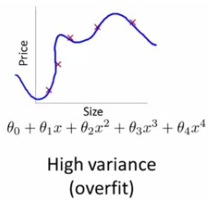泰勒公式

欲理解多项式函数的过拟合，必先理解泰勒公式。

泰勒公式是一种计算近似值的方法，它是一个用函数某点的信息描述在该点附近取值的公式。已知函数在某一点的各阶导数值的情况之下，泰勒公式可以用这些导数值做系数构建一个多项式来逼近函数在这一点的邻域中的值。

如果f(x)在x0处具有任意阶导数，那么泰勒公式是这样的：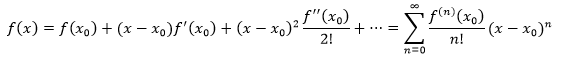上式中的幂级数称为f(x)在x0点的泰勒级数。（0的阶乘是1）

更多泰勒公式的介绍可参考  单变量微积分笔记31——幂级数和泰勒级数

泰勒公式的应用

来看一个泰勒公式的应用。假设一个小偷盗取了一辆汽车，他在高速公路上沿着一个方向行驶，车辆的位移s是关于时间t的函数。警方接到报案后马上调取监控，得知在零点（t=0时刻）小偷距车辆丢失地点的位移是s0。现在的时间是0:30，警方想要在前方设卡，从而能在凌晨1点拦住小偷，应该在哪里设卡呢？

我们知道车辆在0点时的位移是s0，现在想要知道凌晨1点时车辆的位置：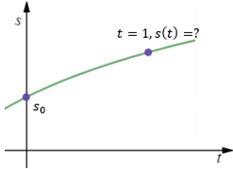可以直接使用泰勒公式：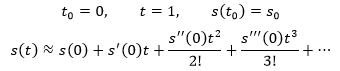泰勒公式可以无限展开，展开得越多，越逼近真实值，并且越到后面的项，对结果的影响越小，我们认为0和1非常接近，所以只展开到2阶导数：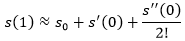这就是最终结果，在此处设卡最有可能在第一时间拦住小偷。

在0点处的泰勒展开

在使用泰勒公式时，经常取x0=0。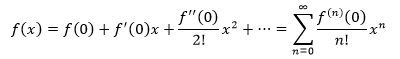f(x)=ex是一个可以用泰勒公式展开的例子，下面是ex在x0=0处的泰勒展开：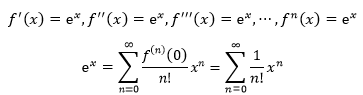当x=1时，还附带得到了e的解释：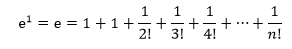我们使用一个很难处理的积分解释泰勒展开的意义，对正态分布进行积分：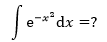常规的方法很难处理。现在，由于被积函数与ex相似，我们又已经知道ex的展开式，所以可以进行下面的变换：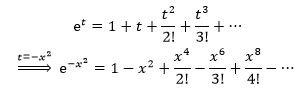将exp(-x2)左右两侧同时积分：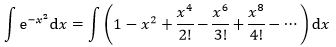很容易计算右侧的每一项积分。

这个例子展示了幂级数展开的意义——把质的困难转化成量的复杂。展开前求解函数的值很困难，展开后是幂级数，虽然有很多很多项，但是每一项都是幂函数，都很容易求解，于是，只要对展开后的函数求和，就能得到展开前的函数的值。

为什么在0点处展开

当x0=0时，可以极大地简化泰勒展开式。之前说泰勒公式是一个用函数某点的信息描述在该点附近取值的公式，一个函数中的某点如果距离0很远怎么办呢？实际上泰勒公式也能够逼近函数在距离0很远处的取值，只不过此时只展开到2阶导数是不够的，需要展开很多项，展开的越多，越能逼近该点。以标准正态分布函数f(x)=exp(-x2)为例，虽然它在二阶展开使与原函数相差较大，但是当展开到40阶时就已经非常接近原函数了。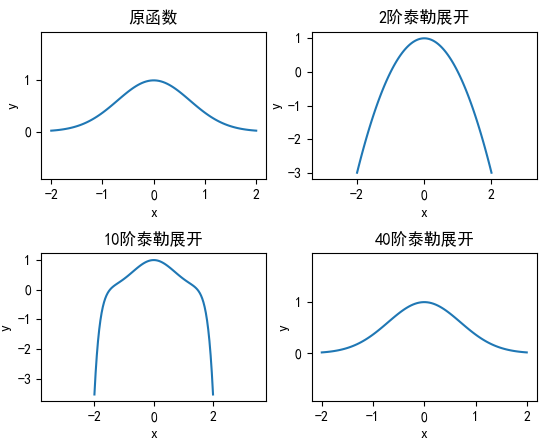多项式函数

理解了泰勒公式后，再回到问题的原点，看看多项式函数为什么可以接近于任何函数。

仍然以标准正态分布为例，它在x0 = 0点处的10阶泰勒展开是：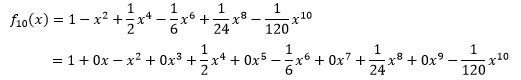如果将每一项中的xi都看作一个维度，那么这个多项式函数可以写成多元线性回归的形式：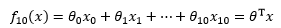这就将一个一元的非线性问题转换成了多元的线性问题，从而利用最小二乘法求得模型参数。

下面的代码以ln(2x) + 2为原函数，生成40个在-1~1之间随机震荡的数据点，并使用线性回归和多项式回归拟合数据点：

import numpy as np
import matplotlib.pyplot as plt

def create_datas():
'''
生成10个待拟合的点
:return: xs, ys
'''
xs = np.arange(0.1, 4, 0.1)
# y = ln(2x) + noize,  -1 <= noize <= 1
ys = np.array([np.log(x * 2) + 2 + np.random.uniform(-1, 1) for x in xs])

return xs, ys

class Regression():
''' 回归类 '''
def __init__(self, xs, ys):
'''
:param xs: 输入数据的特征集合
:param ys: 输入数据的标签集合
'''
self.xs, self.ys = xs, ys
self.theta = None # 模型参数

def train_datas(self, xs=None):
'''
重新构造训练样本的特征和标签
:param xs: 输入数据的特征集合
:return: 矩阵形式的训练样本特征和标签
'''
xs = self.xs if xs is None else xs
X = self.train_datas_x(xs)
Y = np.c_[ys] # 将ys转换为m行1列的矩阵
return X, Y

def train_datas_x(self, xs):
'''
重新构造训练样本的特征
:param xs: 输入数据的特征集合
:return: 矩阵形式的训练样本特征
'''
m = len(xs)
# 在第一列添加x0,x0=1,并将二维列表转换为矩阵
X = np.mat(np.c_[np.ones(m), xs])
return X

def fit(self):
''' 数据拟合 '''
X, Y = self.train_datas()
self.theta = (X.T * X).I * X.T * Y

def predict(self, xs):
'''
根据模型预测结果
:param xs: 输入数据的特征集合
:return: 预测结果
'''
X = self.train_datas(xs=xs)
return self.theta.T * X.T

def show(self):
''' 绘制拟合结果 '''
plt.figure()
plt.scatter(self.xs, self.ys, color='r', marker='.', s=10)  # 绘制数据点
self.show_curve(plt) # 绘制函数曲线
plt.xlabel('x')
plt.ylabel('y')
plt.axis('equal')
plt.rcParams['font.sans-serif'] = ['SimHei']  # 用来正常显示中文标签
plt.rcParams['axes.unicode_minus'] = False  # 解决中文下的坐标轴负号显示问题
plt.legend(['拟合曲线', '样本点'])
plt.show()

def show_curve(self, plt):
''' 绘制函数曲线 '''
pass

def global_fun(self):
''' 返回目标函数 '''
gf = ['(' + str(t[0, 0]) + str(i) + ')x^' + str(i) for i, t in enumerate(self.theta)]
return ' + '.join(gf)

class Linear(Regression):
''' 线性模型'''
def show_curve(self, plt):
'''
绘制拟合结果
:param plt: 输入数据的特征集合
'''
xx = [self.xs, self.xs[-1]]
yy = self.predict(xx)
plt.plot(xx, np.array(yy))

class Multinomial(Regression):
''' 多项式回归模型 '''
def __init__(self, xs, ys, n=3):
'''
:param xs: 输入数据的特征集合
:param ys: 输入数据的标签集合
:param n: 多项式的项数
'''
super().__init__(xs, ys)
self.n = n

def train_datas_x(self, xs):
'''
重新构造训练样本的特征
:param xs: 输入数据的特征集合
:return: 矩阵形式的训练样本特征
'''
X = super().train_datas_x(xs)
for i in range(2, self.n + 1):
X = np.column_stack((X, np.c_[xs ** i])) # 构造样本的其他特征
return X

def show_curve(self, plt):
''' 绘制函数曲线 '''
xx = np.linspace(self.xs, self.xs[-1], len(self.xs) * 20)
yy = self.predict(xx)
plt.plot(xx, np.array(yy), '-')

if __name__ == '__main__':
xs, ys = create_datas()
regressions = [Linear(xs, ys), Multinomial(xs, ys), Multinomial(xs, ys, n=5), Multinomial(xs, ys, n=10)]
for r in regressions:
r.fit()
r.show()
print(r.global_fun())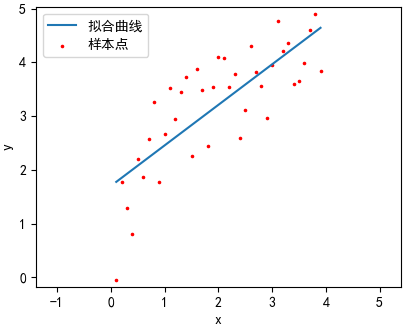(1.702537204930)x^0 + (0.75431357262260011)x^1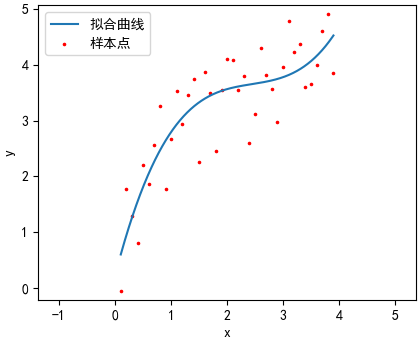(0.23422131704216660)x^0 + (3.8713793437217621)x^1 + (-1.51749485964066682)x^2 + (0.206815637166500283)x^3(0.0023811193415048670)x^0 + (4.707160334405161)x^1 + (-2.03334533257402762)x^2 + (0.095635349482284143)x^3 + (0.130611330518000564)x^4 + (-0.021122013844903465)x^5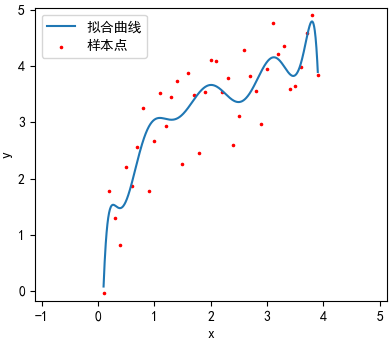(-4.7285135624557920)x^0 + (77.637488456533421)x^1 + (-377.238590224254552)x^2 + (932.32693158635363)x^3

+ (-1305.30725871564164)x^4 + (1112.9257341435945)x^5 + (-598.57958115210336)x^6

+ (203.91275172701427)x^7 + (-42.641981259587898)x^8 + (4.9915417588645349)x^9 + (-0.250300601937088710)x^10

看来第二、三条曲线的拟合效果比较好，第一幅图欠拟合，四过拟合。

作者：我是8位的

出处：http://www.cnblogs.com/bigmonkey

本文以学习、研究和分享为主，如需转载，请联系本人，标明作者和出处，非商业用途！

扫描二维码关注公作者众号“我是8位的”展开全文• 这里我们主要考虑用多项式函数去进行曲线拟合 （1）polyfit 函数 功能： 求得结果为最小二乘法拟合的多项式系数。 格式： p = polyfit(X,Y,n); X,Y为进行拟合的样本数据，类型为矩阵。n为拟合的多项式次数，看散点...
目录一.拟合所需函数（1）polyfit 函数（2）polyval 函数二.举例（预测人口）（1）问题数据（2）求解（3）完整代码（4）注意点
一.拟合所需函数
这里我们主要考虑用多项式函数去进行曲线拟合
（1）polyfit 函数
功能： 求得结果为最小二乘法拟合的多项式系数。
格式：

p = polyfit(X,Y,n);

X,Y为进行拟合的样本数据，类型为矩阵。n为拟合的多项式次数，看散点图判断拟合次数。p为拟合后返回的多项式系数矩阵。

[p,s] = polyfit(X,Y,n);

返回两个结果，s为采样点的误差数据

[p,s,mu] = polyfit(X,Y,n);

返回三个结果，mu是一个二元向量，其中mu(1)是X的平均值即mean(X)， mu(2)是X的标准差即std(X)。

（2）polyval 函数
功能： 求得在上面函数所得的p系数下，某一点预测的函数值。
格式：

polyval(p,15);

返回值为X=15时的函数值。

二.举例（预测人口）
（1）问题数据

某地区2010-2020人口数据：

x
2010
2011
2012
2013
2014
2015
2016
2017
2018
2019
2020

y
3.9
5.3
7.2
9.6
12.9
17.1
23.2
31.4
38.6
50.2
63.0

（2）求解
我们先分步求解，后面有完整代码
【1】先查看散点图趋势
x=2010:1:2020;
y=[3.9,5.3,7.2,9.6,12.9,17.1,23.2,31.4,38.6, 50.2,63.0];
plot(x,y,'*');【2】进行多项式函数拟合(这里可以尝试不同的次数择优选取)
p = polyfit(x,y,2);
plot(x,y,'*',x,polyval(p,x))可以看到结果基本吻合

【3】可以继续进行预测未来的人口
polyval(p,2021)

ans =

74.4776

（3）完整代码
x=2010:1:2020;
y=[3.9,5.3,7.2,9.6,12.9,17.1,23.2,31.4,38.6, 50.2,63.0];
p = polyfit(x,y,2);
plot(x,y,'*',x,polyval(p,x))
polyval(p,2021);

（4）注意点

我们在选取数据时，并不是数据越多越好。

在选取数据时，同时要考虑到实际情况，就比如说对人口预测，可能前10年发生了天灾人祸使得前十年的数据严重不符，那么这一阶段的数据就可以舍弃。

曲线拟合作用

预测趋势

总结规律

估算数据


展开全文• 在本笔记中，我们将从简单易懂的多项式函数拟合实验出发，谈一谈如今做机器学习绕不开的三个重要概念：模型选择、欠拟合和过拟合，并且进一步挖掘如何选择模型、如何避免欠拟合和过拟合问题。本笔记主要从下面 ——...
在本笔记中，我们将从简单易懂的多项式函数拟合实验出发，谈一谈如今做机器学习绕不开的三个重要概念：模型选择、欠拟合和过拟合，并且进一步挖掘如何选择模型、如何避免欠拟合和过拟合问题。本笔记主要从下面五个方面展开：

文章目录1 模型选择1.1 训练误差和泛化误差1.2 模型选择2 欠拟合和过拟合2.1 模型复杂度2.2 训练数据集大小3 多项式函数拟合实验3.1 三阶多项式函数拟合（正常）3.2 线性函数拟合（欠拟合）3.3 训练样本不足（过拟合）3.4 小结4 提高模型泛化能力的两种方法4.1 权重衰减4.2 丢弃法5 心得体会

1 模型选择
1.1 训练误差和泛化误差
首先，我们需要区分训练误差（training error）和泛化误差（generalization error）。通俗来讲，前者指模型在训练数据集上表现出的误差，后者指模型在任意一个测试数据样本上表现出的误差的期望，并常常通过测试数据集上的误差来近似。计算训练误差和泛化误差可以使用之前介绍过的损失函数，例如线性回归用到的平方损失函数和softmax回归用到的交叉熵损失函数。
让我们以高考为例来直观地解释训练误差和泛化误差这两个概念。训练误差可以认为是做往年高考试题（训练题）时的错误率，泛化误差则可以通过真正参加高考（测试题）时的答题错误率来近似。假设训练题和测试题都随机采样于一个未知的依照相同考纲的巨大试题库。如果让一名未学习中学知识的小学生去答题，那么测试题和训练题的答题错误率可能很相近。但如果换成一名反复练习训练题的高三备考生答题，即使在训练题上做到了错误率为0，也不代表真实的高考成绩会如此。
在机器学习里，我们通常假设训练数据集（训练题）和测试数据集（测试题）里的每一个样本都是从同一个概率分布中相互独立地生成的。基于该独立同分布假设，给定任意一个机器学习模型（含参数），它的训练误差的期望和泛化误差都是一样的。例如，如果我们将模型参数设成随机值（小学生），那么训练误差和泛化误差会非常相近。但我们从前面几节中已经了解到，模型的参数是通过在训练数据集上训练模型而学习出的，参数的选择依据了最小化训练误差（高三备考生）。所以，训练误差的期望小于或等于泛化误差。也就是说，一般情况下，由训练数据集学到的模型参数会使模型在训练数据集上的表现优于或等于在测试数据集上的表现。由于无法从训练误差估计泛化误差，一味地降低训练误差并不意味着泛化误差一定会降低。
机器学习模型应关注降低泛化误差。
1.2 模型选择
在机器学习中，通常需要评估若干候选模型的表现并从中选择模型。这一过程称为模型选择（model selection）。可供选择的候选模型可以是有着不同超参数的同类模型。以多层感知机为例，我们可以选择隐藏层的个数，以及每个隐藏层中隐藏单元个数和激活函数。为了得到有效的模型，我们通常要在模型选择上下一番功夫。下面，我们来描述模型选择中经常使用的验证数据集（validation data set）。

验证数据集

从严格意义上讲，测试集只能在所有超参数和模型参数选定后使用一次。不可以使用测试数据选择模型，如调参。由于无法从训练误差估计泛化误差，因此也不应只依赖训练数据选择模型。鉴于此，我们可以预留一部分在训练数据集和测试数据集以外的数据来进行模型选择。这部分数据被称为验证数据集，简称验证集（validation set）。例如，我们可以从给定的训练集中随机选取一小部分作为验证集，而将剩余部分作为真正的训练集。
然而在实际应用中，由于数据不容易获取，测试数据极少只使用一次就丢弃。因此，实践中验证数据集和测试数据集的界限可能比较模糊。从严格意义上讲，除非明确说明，否则本书中实验所使用的测试集应为验证集，实验报告的测试结果（如测试准确率）应为验证结果（如验证准确率）。

$k$折交叉验证

由于验证数据集不参与模型训练，当训练数据不够用时，预留大量的验证数据显得太奢侈。一种改善的方法是$k$折交叉验证（$k$-fold cross-validation）。在$k$折交叉验证中，我们把原始训练数据集分割成$k$个不重合的子数据集，然后我们做$k$次模型训练和验证。每一次，我们使用一个子数据集验证模型，并使用其他$k-1$个子数据集来训练模型。在这$k$次训练和验证中，每次用来验证模型的子数据集都不同。最后，我们对这$k$次训练误差和验证误差分别求平均。

2 欠拟合和过拟合
接下来，我们将探究模型训练中经常出现的两类典型问题：一类是模型无法得到较低的训练误差，我们将这一现象称作欠拟合（underfitting）；另一类是模型的训练误差远小于它在测试数据集上的误差，我们称该现象为过拟合（overfitting）。在实践中，我们要尽可能同时应对欠拟合和过拟合。虽然有很多因素可能导致这两种拟合问题，在这里我们重点讨论两个因素：模型复杂度和训练数据集大小。
2.1 模型复杂度
为了解释模型复杂度，我们以多项式函数拟合为例。给定一个由标量数据特征$x$和对应的标量标签$y$组成的训练数据集，多项式函数拟合的目标是找一个$K$阶多项式函数$\hat{y} = b + \sum_{k=1}^K x^k w_k$来近似 $y$。在上式中，$w_k$是模型的权重参数，$b$是偏差参数。与线性回归相同，多项式函数拟合也使用平方损失函数。特别地，一阶多项式函数拟合又叫线性函数拟合。
因为高阶多项式函数模型参数更多，模型函数的选择空间更大，所以高阶多项式函数比低阶多项式函数的复杂度更高。因此，高阶多项式函数比低阶多项式函数更容易在相同的训练数据集上得到更低的训练误差。给定训练数据集，模型复杂度和误差之间的关系通常如图1所示。给定训练数据集，如果模型的复杂度过低，很容易出现欠拟合；如果模型复杂度过高，很容易出现过拟合。应对欠拟合和过拟合的一个办法是针对数据集选择合适复杂度的模型。图1 模型复杂度对欠拟合和过拟合的影响
2.2 训练数据集大小
影响欠拟合和过拟合的另一个重要因素是训练数据集的大小。一般来说，如果训练数据集中样本数过少，特别是比模型参数数量（按元素计）更少时，过拟合更容易发生。此外，泛化误差不会随训练数据集里样本数量增加而增大。因此，在计算资源允许的范围之内，我们通常希望训练数据集大一些，特别是在模型复杂度较高时，例如层数较多的深度学习模型。

3 多项式函数拟合实验
为了理解模型复杂度和训练数据集大小对欠拟合和过拟合的影响，下面我们以多项式函数拟合为例来实验。本次实验也是基于PyTorch来完成，正好学习理论的同时进一步对深度学习代码进行学习。
首先导入实验需要的包或模块。
import torch
import numpy as np
from matplotlib import pyplot as plt

我们将生成一个人工数据集。在训练数据集和测试数据集中，给定样本特征$x$，我们使用如下的三阶多项式函数来生成该样本的标签：
$y = 1.2x - 3.4x^2 + 5.6x^3 + 5 + \epsilon$其中噪声项$\epsilon$服从均值为0、方差为0.01的正态分布。训练数据集和测试数据集的样本数都设为100。
n_train, n_test, true_w, true_b = 100, 100, [1.2, -3.4, 5.6], 5
features = torch.randn((n_train + n_test, 1))
poly_features = torch.cat((features, torch.pow(features, 2), torch.pow(features, 3)), 1)
labels = (true_w * poly_features[:, 0] + true_w * poly_features[:, 1]
+ true_w * poly_features[:, 2] + true_b)
labels += torch.tensor(np.random.normal(0, 0.01, size=labels.size()), dtype=torch.float)


torch.randn(size)表示从标准正态分布中随机抽出一组数组成一个size大小的张量。如果要自定义正态分布的均值和方差可以利用torch.tensor(np.random.normal(0, 0.01, size=labels.size())）先利用numpy内置函数再转成tensor。
torch.cat看到函数的第一印象还以为是“张量猫”，但是其真正含义为张量拼接，最后一个key之前的参数都是待拼接的张量。最后为“0”表示按行拼接，即“越拼越深”；“1”表示按列拼接，即“越拼越长”。这个地方很巧妙的一点就是将一次项、二次项以及三次项放在一个张量中方便调用。

然后我们先定义作图函数semilogy，其中$y$轴使用了对数尺度。
def semilogy(x_vals, y_vals, x_label, y_label, x2_vals, y2_vals, legend):
plt.xlabel(x_label)
plt.ylabel(y_label)
plt.semilogy(x_vals, y_vals)
if x2_vals and y2_vals:
plt.semilogy(x2_vals, y2_vals, linestyle=':')
plt.legend(legend)


plt.semilogy表示将y轴按照对数输出，x轴不变。画图的函数后面隐藏的key会很多，所以经常看到会不知道是什么意思，这个时候需要多看多积累。
if x2_vals and y2_vals:肯定会有很多读者会问为什么要加这一句，其实这一句的意思是如果第二个图为空，也就不需要画图显示图例了。

和线性回归一样，多项式函数拟合也使用平方损失函数。因为我们将尝试使用不同复杂度的模型来拟合生成的数据集，所以我们把模型定义部分放在fit_and_plot函数中。
num_epochs, loss = 100, torch.nn.MSELoss()

def fit_and_plot(train_features, test_features, train_labels, test_labels):
net = torch.nn.Linear(train_features.shape[-1], 1)
# 通过Linear文档可知，pytorch已经将参数初始化了，所以我们这里就不手动初始化了

batch_size = min(10, train_labels.shape)
dataset = torch.utils.data.TensorDataset(train_features, train_labels)

optimizer = torch.optim.SGD(net.parameters(), lr=0.01)
train_ls, test_ls = [], []
for _ in range(num_epochs):
for X, y in train_iter:
l = loss(net(X), y.view(-1, 1))
l.backward()
optimizer.step()
train_labels = train_labels.view(-1, 1)
test_labels = test_labels.view(-1, 1)
train_ls.append(loss(net(train_features), train_labels).item())
test_ls.append(loss(net(test_features), test_labels).item())
print('final epoch: train loss', train_ls[-1], 'test loss', test_ls[-1])
semilogy(range(1, num_epochs + 1), train_ls, 'epochs', 'loss',
range(1, num_epochs + 1), test_ls, ['train', 'test'])
print('weight:', net.weight.data,
'\nbias:', net.bias.data)


l = loss(net(X), y.view(-1, 1))这个地方要注意的是损失函数中传入的两个参数必须同型，计算的时候要注意。

3.1 三阶多项式函数拟合（正常）
我们先使用与数据生成函数同阶的三阶多项式函数拟合。实验表明，这个模型的训练误差和在测试数据集的误差都较低。训练出的模型参数也接近真实值：$w_1 = 1.2, w_2=-3.4, w_3=5.6, b = 5$。
fit_and_plot(poly_features[:n_train,:],poly_features[n_train:,:],labels[:n_train],labels[n_train:])

输出：图2 正常情况下的输出
这里还要额外插一句，就是这个实验我觉得设计得很巧妙，就是将多项式中的系数当成神经网络中的参数，而将多项式中的常数项当成神经网络中的偏置项，这样一来就是可以保证我的输入为3个未知数，方便理解，要不然就要再加一个神经元并且这个神经元的输入恒为1，用4个参数并且不带偏置项的线性层来解决。
3.2 线性函数拟合（欠拟合）
我们再试试线性函数拟合。很明显，该模型的训练误差在迭代早期下降后便很难继续降低。在完成最后一次迭代周期后，训练误差依旧很高。线性模型在非线性模型（如三阶多项式函数）生成的数据集上容易欠拟合，即在训练数据集上都不能达到一个很小的误差。
fit_and_plot(features[:n_train,:],features[n_train:,:],labels[:n_train],labels[n_train:])

输出：图3 欠拟合情况下的输出
3.3 训练样本不足（过拟合）
事实上，即便使用与数据生成模型同阶的三阶多项式函数模型，如果训练样本不足，该模型依然容易过拟合。让我们只使用两个样本来训练模型。显然，训练样本过少了，甚至少于模型参数的数量。这使模型显得过于复杂，以至于容易被训练数据中的噪声影响。在迭代过程中，尽管训练误差较低，但是测试数据集上的误差却很高。这是典型的过拟合现象。
fit_and_plot(poly_features[0:2,:],poly_features[n_train:,:],labels[0:2],labels[n_train:])

输出：图4 过拟合情况下的输出
3.4 小结

由于无法从训练误差估计泛化误差，一味地降低训练误差并不意味着泛化误差一定会降低。机器学习模型应关注降低泛化误差。
可以使用验证数据集来进行模型选择。
欠拟合指模型无法得到较低的训练误差，过拟合指模型的训练误差远小于它在测试数据集上的误差。
应选择复杂度合适的模型并避免使用过少的训练样本。

4 提高模型泛化能力的两种方法
本节主要介绍应对过拟合问题，提高模型泛化性能的两种常用方法：权重衰减(weight decay)和丢弃法(dropout)。
4.1 权重衰减
权重衰减等价于$L_2$范数正则化(regularization)。正则化通过为模型损失函数添加惩罚项使学出的模型参数值较小，是应对过拟合的常用手段。我们先描述$L_2$范数正则化，再解释它为何又称权重衰减。
$L_2$范数正则化在模型原损失函数基础上添加$L_2$范数惩罚项，从而得到训练所需要最小化的函数。$L_2$范数惩罚项指的是模型权重参数每个元素的平方和与一个正的常数的乘积。以线性回归中的线性回归损失函数
$\ell(w_1, w_2, b) = \frac{1}{n} \sum_{i=1}^n \frac{1}{2}\left(x_1^{(i)} w_1 + x_2^{(i)} w_2 + b - y^{(i)}\right)^2$为例，其中$w_1, w_2$是权重参数，$b$是偏差参数，样本$i$的输入为$x_1^{(i)}, x_2^{(i)}$，标签为$y^{(i)}$，样本数为$n$。将权重参数用向量$\boldsymbol{w} = [w_1, w_2]$表示，带有$L_2$范数惩罚项的新损失函数为
$\ell(w_1, w_2, b) + \frac{\lambda}{2n} |\boldsymbol{w}|^2,$其中超参数$\lambda > 0$。当权重参数均为0时，惩罚项最小。当$\lambda$较大时，惩罚项在损失函数中的比重较大，这通常会使学到的权重参数的元素较接近0。当$\lambda$设为0时，惩罚项完全不起作用。上式中$L_2$范数平方$|\boldsymbol{w}|^2$展开后得到$w_1^2 + w_2^2$。
个人理解：权重衰减其实质上就是在降低模型的复杂度。举个例子来说，如果我现在要拟合几个固定的点，我可以选择低次函数，也可以选择高次函数，如果次数太高那么他的泛化能力肯定差，对于高次函数而言他之所以泛化性能大直观上而言就是波动弯曲起伏太大不具有一般泛化能力，所以这就代表着其每一点附近的梯度会比较大，那么就导致这个高次函数的系数很大，所以现在权重衰减就是要强行限制这个系数，不让系数变大这样就会防止学出来的模型太复杂，避免泛化能力较低。（之前一直不理解为什么要让权重变小，这么一解释是不是清晰了许多）
具体实现方法就是：在初始化网络的时候分别对权重和偏置进行初始化，然后对他们分别设置优化器optimizer，优化器中有一个key就是代表的是否进行权重衰减，代码如下：
nn.init.normal_(net.weight, mean=0, std=1)
nn.init.normal_(net.bias, mean=0, std=1)

optimizer_w = torch.optim.SGD(params=[net.weight],lr,weight_decay=wd) #对权重参数衰减
optimizer_b = torch.optim.SGD(params=[net.bias],lr)  #不对偏差参数衰减

4.2 丢弃法图5 隐藏层使用了丢弃法的多层感知机
图5描述了一个单隐藏层的多层感知机。其中输入个数为4，隐藏单元个数为5，且隐藏单元$h_i$（$i=1, \ldots, 5$）的计算表达式为
$h_i = \phi\left(x_1 w_{1i} + x_2 w_{2i} + x_3 w_{3i} + x_4 w_{4i} + b_i\right)$这里$\phi$是激活函数，$x_1, \ldots, x_4$是输入，隐藏单元$i$的权重参数为$w_{1i}, \ldots, w_{4i}$，偏差参数为$b_i$。当对该隐藏层使用丢弃法时，其中$h_2$和$h_5$被清零。这时输出值的计算不再依赖$h_2$和$h_5$，在反向传播时，与这两个隐藏单元相关的权重的梯度均为0。由于在训练中隐藏层神经元的丢弃是随机的，即$h_1, \ldots, h_5$都有可能被清零，输出层的计算无法过度依赖$h_1, \ldots, h_5$中的任一个，从而在训练模型时起到正则化的作用，并可以用来应对过拟合。
在测试模型时，我们为了拿到更加确定性的结果，一般不使用丢弃法，所以一般测试之前都会用eval()来关闭dropout的功能。
具体实现方法就是：在需要使用dropout的地方加入nn.Dropout(P)这一层或者利用F.Dropout(P)这一函数来完成这一功能，括号中的P表示置零神经元的概率，代码如下：
net = nn.Sequential(
nn.Linear(num_inputs, num_hiddens1),
nn.ReLU(),
nn.Dropout(drop_prob1),
nn.Linear(num_hiddens1, num_hiddens2),
nn.ReLU(),
nn.Dropout(drop_prob2),
nn.Linear(num_hiddens2, 10)
)

当然这个Dropout层放置的位置也可以好好研究一下。

5 心得体会

在上一篇笔记中主要讲到了softmax分类在MLP中的应用，这一次的笔记主要围绕模型的结果及泛化能力改进进行了一些讨论。
无论搭建什么模型，我们的最终目的是它有一个很好的泛化能力，可以对于未知的数据有一个很好的表现，而绝不仅仅在于训练的好与坏。
这之间的关系就好像一个博弈，为了提高测试的准确度其实有时放大训练误差也未尝不可。


展开全文机器学习 深度学习 神经网络 正则化 人工智能
• 全域多项式插值指的是在整个插值区域内形成一个多项式函数作为插值函数。关于多项式插值的基本知识，见“计算基本理论”。 　在单项式基插值和牛顿插值形成的表达式中，求该表达式在某一点处的值使用的Horner嵌套...
• 线性回归模型的优化目标函数by Björn Hartmann 比约恩·哈特曼(BjörnHartmann) When reading articles about machine learning, I often suspect that authors misunderstand the term “linear model.” Many ...python 机器学习 人工智能 大数据 深度学习
• ## 插值多项式例子

千次阅读 2017-12-02 14:15:30
1. Hermite 插值多项式例子 给定函数 exe^x 在区间 [−1,1][-1,1] 上的数据表如下： xx -1 0 1 f(x)f(x) 0.3679 1.0000 2.7182 f′(x)f'(x) 0.3679 1.0000 2.7182 给出 Hermite 插值多项式，并计算其...插值法
• 本代码主要利用MATLAB工具实现MATLAB——多项式加法函数示例，简单明了，易于理解
• 多维多项式插值和逼近工具 - polymake.m 计算多项式插值系数。 - polyeval.m 计算给定点的多项式。 - polycomb.m 支持功能。... 有关更多详细信息，请查看示例文件 pexample.m 或查看函数 polymake.m 的帮助文本matlab
• 整理自《C常用算法程序集》 作者 徐士良 清华大学出版社 8.1最小二乘曲线拟合 ... 表示3次多项式; x作为数据点之外的一个自变量，其对应的函数值： y = (int)(A+A*x+A*x*x+A*x*x*x);C 最小二乘 曲线拟合
• polyfit函数是matlab中用于进行曲线拟合的一个函数。其数学基础是最小二乘法曲线拟合原理。曲线拟合：已知离散点上的数据集，即已知在点集上的函数值，构造一个解析函数（其图形为一曲线）使在原离散点上尽可能接近...
• %分别用分段线性插值、此次方程式插值和样条插值确定插值函数并进行比较 %月儿半弯 x=0:10; y=cos(x); xi=0:.25:10; y0=cos(xi); %精确值 y1=interp1(x,y,xi); %线性插值结果 y2=interp1(x,y,xi,'cubic'); %三次方程...
• ## 多项式

千次阅读 2013-09-17 14:18:32
在数学领域里，多项式是由变量以及标量（一般是实数或复数）经乘法及加法构法而成，属于整式的代数式。下列四种都是多项式多项式中每一个 x n 皆称之为多项式的项 次数：多项式 x n 中每一项的...非多项式例子数学 算法
• #多项式回归模型 import torch import numpy as np from torch.autograd import Variable import matplotlib.pyplot as plt torch.manual_seed(2018) # 定义一个多变量函数 w_target = np.array([0.5, 3, 2.4]) # ...
• Matlab多项式 ...先来看下例子： 加减运算没什么难度，之间看乘除的表示： syms f g x; f=3*x^2+2*x+2; g=3*x+1; ans1=f*g; ans2=f/g; disp(ans1);disp(ans2) 结果如下： (3x + 1)(3x^2 + 2x + 2) (3matlab 线性代数 算法
• 多项式拟合(最小二乘法) a基本原理： b拟合函数原理： c方法优缺点： 优点： 思想简单，实现容易。... 需要做预测的话需要数据大致满足多项式函数。 d算法入口： 该方法主要用到的函数是n..python 机器学习
• 生成函数是说，构造这么一个多项式函数 g(x) ，使得 x 的 n 次方系数为 f(n) 。 对于母函数，看到最多的是这样两句话： 1.“ 把组合问题的加法法则和幂级数的乘幂对应起来。 ” 2.“ 把离散数列和幂级数一...
• 多项式分布由二项式分布推广而来，二项式分布的最简单例子是抛硬币，抛完之后硬币出现正反面的概率。在此之前回忆一下二项式，二项式...常见的那个是多项式函数，多项式就是最简单的那个。像投硬币一样，也就是正反...
• matlab开发-fit多维多项式。这个例子适用于CMYK和LAB之间，它假定LAB是CMYK的多项式函数
• 1.1.16.多项式回归：基函数拓展线性回归模型机器学习中一种常见模式是使用线性模型去训练非线性模型。这种方式保持了常见线性方法的快速性，同时适用于更广泛的数据。例如，一个简单的线性回归可以通过系数拓展到...
• 多项式拟合： x=1:10; y=randn(size(x)); p=polyfit(x,y,7); % 7阶多项式，返回拟合多项式的降幂系数 x1=0:.1:10; y_hat=polyval(p,x1); plot(x1,y_hat,x,y,'r*') 或者直接使用matlab的拟合工具箱 写好x, y值 x=1:...
• % 聚合物% 此函数将 n 阶多项式拟合到给定的数据点 (x,y) % 使用回归技术% ----------------------------------------------- % 输入% xx 点% yf(x) % n 多项式阶数； 1 为线性% ----------------------------------...matlab
• 后期更新：matlab中产生m序列最简单的方法是调用idinput()函数，关于idinput()的具体用法可以在matlab中查看说明，这里举两个简单的例子： src = idinput(8191,'RBS')'; % 产生随机的二进制数据 1 -1 m = idinput...
• 机器学习中的一个常见模式是使用数据的非线性函数训练的线性模型。 这种方法保持了线性方法的一般快速的性能，同时允许它们适应更宽范围的数据。 例如，可以通过从系数构建多项式特征来扩展简单的线性回归。 在标准...python 机器学习
• matlab 的 pchip 和 spline 函数适用于期刊案例：perpchip 和 perspline。 给出了一些例子matlab
• C 语言 clock() 函数，例：计算多项式值 /** * clock(): 捕捉从程序开始运行到 clock() 被调用时所耗费的时间。 * 这个时间单位是 clock tick, 即“时钟打点”。 * 常数 CLK_TCK: 机器时钟每秒所走的始终打点数。...
• 多项式回归:用基函数扩展线性模型 机器学习中的一种常见模式是使用在数据的非线性函数上训练的线性模型。这种方法保持了线性方法的快速性能，同时允许它们拟合范围更广的数据。 例如，一个简单的线性回归可以由系数...python 机器学习
• n = n 次多项式的项数，从 0 开始。 输出变量： M = 结果表 {'Iter', 'approx', 'et', 'ea'}; 大约=乐趣的近似最终值。 etf = 最终百分比相对误差。 eaf = 最终近似百分比误差。 例子： [approx, ...matlab
• 转自：https://blog.csdn.net/It_BeeCoder/article/details/78779385在运行MATLAB...所以，工具箱拟合结果十分不适合调用，以及继续下面的操作，所以我们需要用到matlab曲线拟合函数,并且以最常用的多项式拟合函数为...matlab polyfit
• 定义 方法 例子 备注 直接建立 p = [1, 2, 3] 代表多项式p = x^2 + 2*x + 3 ...多项式函数 功能 例子 备注 计算多项式的值 polyval(p, b) 计算x = b的时候的多项式的值, b可以是矩阵相当于点运算 求matlab...